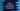# 3 ways to concatenate tuple elements by delimeter in Python## How to concatenate tuple elements by delimeter in Python:

Tuples are used in python to store a collection of different python objects separated by comma. Tuple is immutable and it is ordered.

For example, the below is a tuple with strings and numbers:

``given_tuple = ('hello', 123, 'world)``

In this post, we will learn how to concatenate the elements of a tuple by using a delimeter. The delimeter can be any string.

## Method 1: By using a loop:

We can use a loop to iterate over the elements of a tuple and join these to a final result string.

The below program does that:

``````given_tuple = ('hello', 123, 'world', True)

final_str = ''
delimeter = '-'

for item in given_tuple:
if not final_str:
final_str += str(item)
else:
final_str += delimeter + str(item)

print(final_str)``````

The idea is that we are iterating through the items in the tuple and for each item, we are converting it to a string and appending it to final_str. final_str is the final result string. This is initialized as empty.

Inside the loop, we are appending the delimeter to the result string only if it is not empty.

If you run this program, it will print the below output:

``hello-123-world-True``

## Method 2: By using Python string join:

join method takes one iterable as the parameter and joins all items in that iterable separating each by a delimeter.

We can use join to join all items of a tuple if all of these items are string. For example, the below program will work:

``````given_tuple = ('hello', 'world', '123')

final_str = ''
delimeter = '-'

final_str = delimeter.join(given_tuple)

print(final_str)``````

It will print:

``hello-world-123``

But, if we have any one non-string value in the tuple, it will throw one exception.

We need to use list comprehension for that:

``````given_tuple = ('hello', 'world', 123, True)

final_str = ''
delimeter = '-'

final_str = delimeter.join([str(item) for item in given_tuple])

print(final_str)``````

We are converting all items to string before calling join. This will give us the required string:

``hello-world-123-True``

## method 3: Using join and map:

This is another way to concatenate the tuple elements. We can use map function to create a map object of strings by converting all elements to string.

We can use join with the delimeter to the result map to create the final result.

``````given_tuple = ('hello', 'world', 123, True)

final_str = ''
delimeter = '-'

final_str = delimeter.join(map(str, given_tuple))

print(final_str)``````

It will print:

``hello-world-123-True``